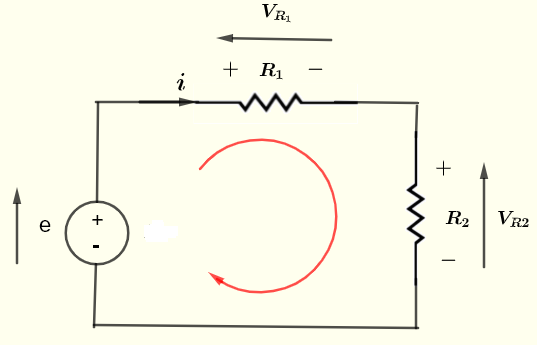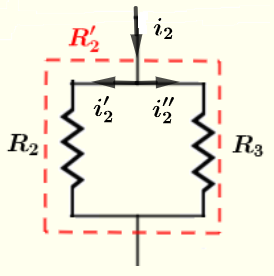# Solve DC Circuits Problems

Kirchhoff's and Ohm's laws are used to solve DC circuits problems.
There are 3 examples; solve in the order that they are presented; this will make it easier to fully understand them.



Problem 1
Find current $i$, voltages $V_{R_1}$ and $V_{R_2}$ in the ciruit below given that the voltage source $e = 20$ Volts, the resistances $R_1 = 100 \; \Omega$ and $R_2 = 300 \; \Omega$.Solution to Problem 1
Apply Kirchhoff's law of voltage to the closed loop in the circle and write the equation
$e - V_{R_1} - V_{R_2} = 0$     (1)
Use Ohm's law to write
$V_{R_1} = i R_1$ and $V_{R_2} = i R_2$
Substitute $V_{R_1}$ and $V_{R_2}$ by their expression in equation (1)
$e - i R_1 - i R_2 = 0$
Rearrange the above so that all term containing $i$ are on one side
$i R_1 + i R_2 = e$
Factor $i$ out
$i ( R_1 + R_2) = e$
Solve for $i$
$i = \dfrac{e}{R_1 + R_2}$
Substitute known quantities
$i = \dfrac{20}{100 + 300} = 0.05$ A
Calculate $V_{R_1}$ and $V_{R_2}$ using Ohm's law
$V_{R_1} = i R_1 = 0.05 \times 100 = 5$ V
$V_{R_2} = i R_2 = 0.05 \times 300 = 15$ V

Problem 2
Given the voltage sources $e_1 = 20$ V, $e_2 = 5$ V, and the resistances $R_1 = 100 \; \Omega$ , $R_2 = 300 \; \Omega$ and $R_3 = 50 \; \Omega$, find all currents through and voltages across the resistors in the circuit.Solution to Problem 2
Kirchhoff's Law of voltage for all three loops $L_1$ , $L_2$ and $L_3$ gives
Loop $L_1$: $e_1 - V_{R_1} - V_{R_2} = 0$      (1)
Loop $L_2$: $V_{R_2} + e_2 - V_{R_3 } = 0$      (2)
Loop $L_3$: $e_1 - V_{R_1} + e_2 - V_{R_3 } = 0$      (3)

Note that if we add equations (1) and (2), we obtain equation (3). Hence any 2 equations from the 3 can be used to solve the given problems. The third one does not give any extra information.
We have 3 unknowns, we therefore need another equation independent from the above equation.
Kirchhoff's Law of current applied at node A gives
$i_1 = i_2 + i_3$      (4)

So we select equations (1), (2) and (4) to write the system of equations
$e_1 - V_{R_1} - V_{R_2} = 0$      (5)
$V_{R_2} + e_2 - V_{R_3 } = 0$      (6)
$i_1 = i_2 + i_3$      (7)

Use Ohm's law to rewrite $V_{R_1}$ and $V_{R_2}$ as follows
$V_{R_1} = R_1 i_1$ and $V_{R_2} = R_2 i_2$
Substitute the above in equations (5) and (6) and rewrite the system of equations (5), (6) and (7) as
$R_1 i_1 + R_2 i_2 = e_1$      (8)
$R_2 i_2 - R_3 i_3 = - e_2$      (9)
$i_1 = i_2 + i_3$      (10)

Substitute the known quantities by their numerical values to obtain a linear system system of equations with three unknowns $i_1$, $i_2$ and $i_3$ .
$100 i_1 + 300 i_2 = 20$      (11)
$300 i_2 - 50 i_3 = - 5$      (12)
$i_1 = i_2 + i_3$      (13)
There are many ways to solve the above system.
One way is to use equation (XIII) and substitute $i_1$ by $i_2 + i_3$ in equations (XI) and (XII) to obtain a system with two unknowns
$100 (i_2 + i_3) + 300 i_2 = 20$      (14)
$300 i_2 - 50 i_3 = - 5$      (15)
Rearrange to rewrite the above system as
$400 i_2 + 100 i_3 = 20$      (16)
$300 i_2 - 50 i_3 = - 5$      (17)
Multiply all terms of equation (17) above by 2 and rewrite the above system as
$400 i_2 + 100 i_3 = 20$      (16)
$600 i_2 - 100 i_3 = - 10$      (17)
Add side by side equations (16) and (17) to obtain one equation in one variable
$1000 i_2 = 10$
Solve for $i_2$
$i_2 = 10/1000 = 0.01$ A
Substitute $i_2$ by $0.01$ in equation (16) and solve for $i_3$
$400 \times 0.01 + 100 i_3 = 20$
Solve for $i_3$
$i_3 = 0.16$ A
Use equation (13) to solve for $i_1$
$i_1 = i_2 + i_3 = 0.01 + 0.16 = 0.17$ A
We now calculate the voltages across the resistors
$V_{R_1} = R_1 I_1 = 100 \times 0.17 = 17$ V
$V_{R_2 } = R_2 I_2 = 300 \times 0.01 = 3$ V
$V_{R_3 } = R_3 I_3 = 50 \times 0.16 = 8$ V

Problem 3
Find all currents through and voltages across the resistors in the circuit below, given the voltage sources $e_1 = 20$ V, $e_2 = 5$ V, and the resistances $R_1 = 100 \; \Omega$ , $R_2 = 120 \; \Omega$, $R_3 = 60 \; \Omega$, $R_4 = 40 \; \Omega$, $R_5 = 240\; \Omega$ and $R_6 = 80 \; \Omega$.Solution to Problem 3
All the details of the solution are presented and in order to make this presentation clear and easy to understand, the solution of this example has 5 parts.

Part 1: Simplify the circuit by grouping resistors
If we apply Kirchhoff's law to the closed loops and nodes in the given circuit above, we will end up with a large number of equations to solve.
However, a quick analysis of the given circuit shows that some of the resistors are
parallel and series configurations as shown below.
Resistors $R_2$ and $R_3$ are in parallel and their equivalent resistance is $R'_2$ as shown in the circuit below.
$R_5$ and $R_6$ are in parallel and their equivalent resistance is in series with $R_4$ and their overall equivalent resistance is $R'_3$ as shown in the circuit below.Use the formula for resistors in parallel to write
$\dfrac{1}{R'_2} = \dfrac{1}{R_2} + \dfrac{1}{R_3}$
Solve the above for $R'_2$ to obtain
$R'_2 = \dfrac{R_2 \cdot R_3}{R_2 + R_3}$
Substitute the knwon quantities to obtain
$R'_2 = \dfrac{120 \cdot 60}{120 + 60} = 40 \; \Omega$
Use formula for resistors in parallel to $R_5$ and $R_6$ and add it to $R_4$ to write $R'_3$ as
$R'_3 = R_4 + \dfrac{R_5 \cdot R_6}{R_5 + R_6}$
Substitute the knwon quantities to obtain
$R'_3 = 40 + \dfrac{240 \cdot 80}{240 + 80} = 100$

Part 2: Calculate $i_1$, $i_2$ and $i_3$ using Kirchhoff's law
We now have a much simpler circuit to solve as shown below.There are 3 unkwons $i_1$, $i_2$ and $i_3$ and we therefore need 3 equations.
Use Kirchhoff's law of current at node A to write
$i_1 = i_2 + i_3$      (1)
Use Use Kirchhoff's law of voltage for the loop on the left
$e_1 - V_{R_1} - V_{R'_2} + e_2 = 0$      (2)
Use Use Kirchhoff's law of voltage for the loop on the right
$- e_2 + V_{R'_2} - V_{R'_3} = 0$      (3)
Use Ohm's law to write
$V_{R_1} = R_1 i_1$
$V_{R'_2} = R'_2 i_2$
$V_{R'_3} = R'_3 i_3$
Substitute the above voltages in equations (2) and (3) and include equation (1) to rewrite the system of 3 equations with 3 unknowns
$e_1 - R_1 i_1 - R'_2 i_2 + e_2 = 0$
$- e_2 + R'_2 i_2 - R'_3 i_3 = 0$
$i_1 = i_2 + i_3$
Substitute the knwon quantities, simplify and rewrite the above system in the form.
$100 i_1 + 40 i_2 = 25$      (4)
$40 i_2 - 100 i_3 = 5$      (5)
$i_1 = i_2 + i_3$      (6)
There are many ways to solve systems of linear equations. Let us use the method of substitution.
Substitute $i_1$ by $i_2 + i_3$ in equations (4) and rewrite equations (4) and (5) as follows
$100 (i_2 + i_3) + 40 i_2 = 25$      (7)
$40 i_2 - 100 i_3 = 5$      (8)
Simplify and group
$140 i_2 + 100 i_3 = 25$
$40 i_2 - 100 i_3 = 5$
Add the above equations to eliminate $i_3$
$180 i_2 = 30$
Solve for $i_2$
$i_2 = 30/180 = 1/6$ A
Use equation (7) (or (8) ) to find $i_3$
$100 i_3 = 25 - 140 i_2$
$i_3 = 1/60$ A
Use equation (6) to find $i_1$
$i_1 = i_2 + i_3 = 1/6 + 1/60 = 11/60$

Part 3: Calculate currents in resistors $R_2$ and $R_3$Apply Kirchhoff's law of current
$i_2'+i_2'' = i_2 = 1/6$
Kirchhoff's law of voltage to the closed loop.
$R_2 i_2' - R_3 i_2'' = 0$
Substitute known quantities in the above equation
$120 i_2' - 60 i_2'' = 0$
Solve the system of 2 equations 2 unknowns
$i_2'+i_2'' = 1/6$
$120 i_2' - 60 i_2'' = 0$
to obtain
$i_2' = 1/18$ A
and $i_2'' = 1/9$ A

Part 4: Calculate currents in resistors $R_5$ and $R_6$
We now calculate the currents through resistors $R_5$ and $R_6$ using the circuit belowApply Kirchhoff's law of current
$i_3'+i_3'' = i_3 = 1/60$
Kirchhoff's law of voltage to the closed loop.
$R_5 i_3' - R_6 i_3'' = 0$
Substitute known quantities in the above equation
$240 i_2' - 80 i_2'' = 0$
Solve the system of 2 equations 2 unknowns
$i_3'+i_3'' = 1/60$
$240 i_2' - 80 i_2'' = 0$
to obtain
$i_3' = 1/240$A
and $i_3''' = 1/80$ A

Part 5: Calculate voltages in all resistors
We now list all resistors and the currecnts through each one of them; and then calculate the voltages across each one of them.
$R_1 = 100 \; \Omega$ , current $i_1 = 11/60$ A     voltage : $V_{R_1} = 100 \times 11/60 = 18.33$ V
$R_2 = 120 \; \Omega$ , current $i_2' = 1/18$ A     voltage : $V_{R_2} = 120 \times 1/18 = 6.67$ V
$R_3 = 60 \; \Omega$ , current $i_2'' = 1/9$A     voltage : $V_{R_3} = 60 \times 1/9 = 6.67$ V
$R_4 = 40 \; \Omega$ , current $i_3 = 1/60$ A     voltage : $V_{R_4} = 40 \times 1/60 = 0.67$ V
$R_5 = 240\; \Omega$ , current $i_3' = 1/240$A     voltage : $V_{R_5} = 240 \times 1/240 = 1$ V
$R_6 = 80 \; \Omega$ , current $i_2'' = 1/80$A     voltage : $V_{R_5} = 80 \times 1/80 = 1$ V